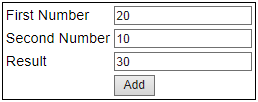Support us .Net Basics C# SQL ASP.NET Aarvi MVC Slides C# Programs Subscribe Download

### Converting strings to numbers in JavaScript

Suggested Videos
Part 6 - Inline vs external javascript
Part 7 - Where should the script tag be placed in html
Part 8 - JavaScript Basics

In this video we will discuss different methods that are available in JavaScript to convert strings to numbers. Let's design the web form as shown below.This form allows the user to enter 2 numbers and add them. Along the way we will learn the use of the following functions
1. parseInt()
2. parseFloat()
3. isNan()

Here is the HTML for the web form
<table style="border:1px solid black; font-family:Arial">
<tr>
<td>First Number</td>
<td><asp:TextBox ID="txtFirstNumber" runat="server"></asp:TextBox></td>
</tr>
<tr>
<td>Second Number</td>
<td><asp:TextBox ID="txtSecondNumber" runat="server"></asp:TextBox></td>
</tr>
<tr>
<td>Result</td>
<td><asp:TextBox ID="txtResult" runat="server"></asp:TextBox></td>
</tr>
<tr>
<td>
</td>
<td>
</td>
</tr>
</table>

Include the following JavaScript in the head section of the web form.
<script type="text/javascript">
{
var firstNumber = document.getElementById("txtFirstNumber").value;
var secondNumber = document.getElementById("txtSecondNumber").value;
document.getElementById("txtResult").value = firstNumber + secondNumber;
}
</script>

Set the onclick attribute of the Add button to call the addNumbers() function. The HTML for the button should be as shown below.

Run the application and enter 20 as the first number and 10 as the second number. Click Add buton. Notice that JavaScript concatenates the numbers instead of adding them. This is because the value property of the textbox is returning the number in a string format.So we need to explicitly do the conversion. This is when parseInt() function is useful. Modify the addNumbers() JavaScript function as shown below.
{
var firstNumber = parseInt(document.getElementById("txtFirstNumber").value);
var secondNumber = parseInt(document.getElementById("txtSecondNumber").value);
document.getElementById("txtResult").value = firstNumber + secondNumber;
}

Run the application and test. Notice that we now get 30 as expected.Let's do another test. Enter 20.5 as the first number and 10.3 as the second number. Click the Add button. Notice that the decimal part is ignored.To retain the decimal places, use parseFloat() function.
{
var firstNumber = parseFloat(document.getElementById("txtFirstNumber").value);
var secondNumber = parseFloat(document.getElementById("txtSecondNumber").value);
document.getElementById("txtResult").value = firstNumber + secondNumber;
}If you leave first number and second number textboxes blank or if you enter text instead of a number, and when you click the Add button, NaN is displayed in result textbox.

NaN in JavaScript stands for Not-a-Number. In JavaScript we have isNaN() function which determines whether a value is an illegal number. This function returns true if the value is not a number, and false if not.

Modify the addNumbers() JavaScript function as shown below.
{
var firstNumber = parseFloat(document.getElementById("txtFirstNumber").value);
if (isNaN(firstNumber))
{
return;
}
var secondNumber = parseFloat(document.getElementById("txtSecondNumber").value);
if (isNaN(secondNumber))
{
return;
}
document.getElementById("txtResult").value = firstNumber + secondNumber;
}

Now, when you leave first number and second number textboxes blank or if you enter text instead of a number, and when you click the Add button, you get relevant validation error messages as expected.

Let's make the validation error message a little more relevant:
If the first number and second number textboxes are left blank, then we want to display the following validation messages
a) First Number is required
b) Second Number is required

If you enter text instead of number
a) Please enter a valid number in the first number textbox
b) Please enter a valid number in the second number textbox

To achieve this modify addNumbers() function as shown below.
{
var firstNumber = document.getElementById("txtFirstNumber").value;
var secondNumber = document.getElementById("txtSecondNumber").value;

if (firstNumber == "")
{
return;
}

firstNumber = parseFloat(firstNumber);
if (isNaN(firstNumber))
{
return;
}

if (secondNumber == "")
{
return;
}

secondNumber = parseFloat(secondNumber)
if (isNaN(secondNumber))
{
return;
}

document.getElementById("txtResult").value = firstNumber + secondNumber;
}1.This comment has been removed by the author.

2.if we add 23abc + 37 its gibing result as 60. is it correct ?

1.Parse Methods will try to get a number from left side and will terminate either at the end of the string or when the first non number value is encountered. That is why 23abc will give 23 on parsing and abc23 will give NaN.
In order to tackle this sitatuon, use Number() method and it will do the trick.
Don't forget to thank Venkat if this comment helps you. (:

3.if we add 10abc + 10 its giving 20.. is it correct ?

1.Correct as long as the intended functionality of parse methods are concerned. But I think this is what you would not want logically, so I would suggest to use Number() method instead.

4.Number method is good instead of using any other method to parse data.

5.i got a doubt on this line " Include the following JavaScript in the head section of the web form".

According to the part 8 video we are supposed to use the script tag just before we close body tag right?

1.thats when you are having external javascript file.This is internal javasript.

It would be great if you can help share these free resources